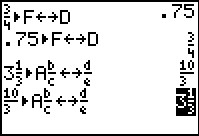# Knowledge Base

## Solution 14240: Converting Between Fractions and Decimals on the TI-73 Explorer™.

### Can the TI-73 Explorer convert a decimal answer to a fraction answer?

The TI-73 Explorer can convert any fraction to a decimal. However when converting a decimal to a fraction, if a fractional equivalent of a decimal does not exist, the calculator returns the same digits.

The calculator also only recognizes and converts (if possible) the first ten digits of any decimal. The Decimal Notation mode determines the display of the result.

The image below depicts some of the conversion options available:Example 1: Converting a fraction to a decimal.
1) Enter 3 and press [b/c] [ 4] [F<->D] [ENTER]

Example 2: Converting a decimal to a fraction.
2) Enter the fraction and press [F<->D] [ENTER]

Example 3: Converting a mixed fraction to an improper fraction.
3) Enter 3 and press [UNIT]  [b/c] [A b/c<->d/e] [ENTER]

Example 4: Converting an improper fraction to a decimal.
4) Press [A b/c<->d/e] [ENTER]

More information on converting between decimals and fractions can be found in the TI-73 Explorer guidebook.